Mobile QR Code1. (School of Electrical and Electronics Engineering, Chung-Ang University, Seoul 06974, Korea)
2. (Research and Development Center, Samsung Display, Yongin 17113, Korea)

Indium-gallium-zinc oxide (IGZO), thin-film transistors (TFTs), quantitative analysis, channel width, self-heating stress

## I. INTRODUCTION

Since the invention of indium gallium zinc oxide (IGZO) thin-film transistors (TFTs) in 2004 by Prof. Hosono and coworkers, IGZO TFTs have attracted considerable attention because of their superior properties including high electron mobility, excellent on/off ratio, low process temperature, and high uniformity [1-4]. These merits render IGZO TFTs particularly suitable for applications in high-resolution organic light emitting diode (OLED) displays . To utilize IGZO TFTs for pixel and gate driver circuits in OLEDs, they should possess high current capability because the drivers will need to handle currents of hundreds of milliamperes on each output [6,7]. To increase the current capability of IGZO TFTs, it is necessary to increase their channel width. Therefore, it is very important to study the effects of channel width on the electrical stability of IGZO TFTs. Previously, some studies have been conducted on the effects of channel width on the electrical stability of IGZO TFTs, especially under self-heating stress (SHS) conditions [8-10]. These studies revealed that the degree of degradation was more significant under SHS conditions in IGZO TFTs with increased channel width. This was mainly attributed to the enhanced self-heating effects in TFTs with a wider channel width, originating from the low thermal conductivity of the IGZO . However, most of these previous studies only analyzed the self-heating effects qualitatively. For example, the dominant degradation mechanism enhanced by the self-heating effects was attributed to one of two mechanisms: electron/hole trapping in the gate dielectric or changes in the subgap density of states (DOS) in the active region [12,13]. However, since several degradation mechanisms of different origins can cause stress-induced electrical performance degradation in IGZO TFTs, it is very important to analyze degradation caused by self-heating quantitatively in IGZO TFTs. In this study, we examined the effects of channel width on electrical performance degradation in commercially-available top-gate self-aligned (TG-SA) coplanar IGZO TFTs under SHS. Furthermore, we comprehensively investigated the quantitative contribution of every degradation mechanism that caused the SHS-induced threshold voltage shift (${\Delta}$V$_{\mathrm{TH}}$) in IGZO TFTs with different channel widths.

## II. EXPERIMENTAL DETAILS

The IGZO TFTs used in this study had a TG-SA coplanar structure and were fabricated using the following process. First, an oxide buffer layer was deposited on a polyimide substrate using plasma-enhanced chemical vapor deposition (PECVD). Then, an IGZO layer (In:Ga:Zn = 1:1:1 at \%) was deposited by radio-frequency magnetron sputtering.

Subsequently, a SiO$_{\mathrm{X}}$ layer was deposited by PECVD as a gate dielectric, followed by the deposition of a gate metal (Molybdenum). After deposition and patterning of the gate electrode and gate dielectric, SiO$_{\mathrm{X}}$ and SiN$_{\mathrm{X}}$ were deposited as an interlayer dielectric (ILD) by PECVD and patterned to form via holes. The via-contact-type n$^{+}$-IGZO source (S) and drain (D) electrodes of Al were then deposited and patterned. Finally, the devices were thermally annealed at 300 $^{\circ}$C in air to achieve stable and uniform electrical performance. The fabricated device is schematically illustrated in Fig. 1. The bias stress experiments were performed on IGZO TFTs with a width (W)/length (L) of 30/5 ${\mu}$m and 50/5 ${\mu}$m. The electrical characterizations were conducted using an Agilent 4156C parameter analyzer. The SHS condition used in this experiment was a gate-to-source voltage (V$_{\mathrm{GS}}$) of 30 V, a drain-to-source voltage (V$_{\mathrm{DS}}$) of 10 V, and a recovery condition of V$_{\mathrm{GS}}$ = V$_{\mathrm{DS}}$ = 0 V at room temperature in a dark environment.

##### Fig. 1. Schematic illustration of fabricated TG-SA coplanar IGZO TFTs.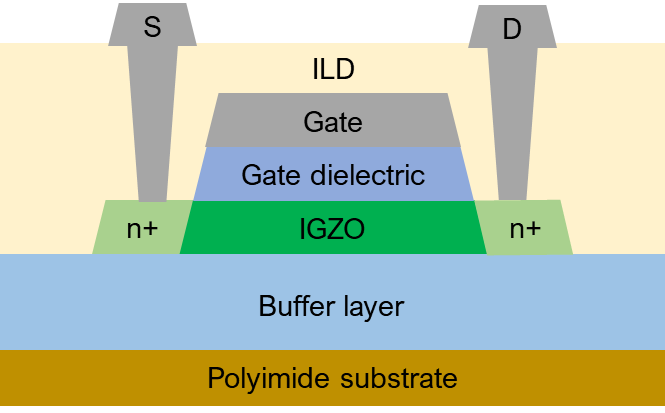## III. RESULTS AND DISCUSSION

Fig. 2(a) and (b) display the time dependencies of the transfer curves measured at V$_{\mathrm{DS}}$ = 0.1 V under SHS for IGZO TFTs with W/L = 30/5 and 50/5 ${\mu}$m, respectively, while Fig. 2(c) and (d) respectively display the transconductances (g$_{\mathrm{m}}$ = ${\mu}$$_{\mathrm{FE}}$${\cdot}$C$_{\mathrm{OX}}$${\cdot}V_{\mathrm{DS}}$${\cdot}$W/L). Here, ${\mu}$$_{\mathrm{FE}} is the field-effect mobility and C_{\mathrm{OX}} is the gate dielectric capacitance per unit area. Fig. 2 demonstrates that the transfer curve shifted in the positive direction and g_{\mathrm{m}} (and {\mu}$$_{\mathrm{FE}}$) increased with increasing stress time in both TFTs. In addition, the degree of transfer curve shift and ${\Delta}$g$_{\mathrm{m}}$ (and ${\Delta}$${\mu}$$_{\mathrm{FE}}$) were larger in the TFT with W/L = 50/5~${\mu}$m.

Fig. 3(a) and (b) display the time dependencies of the transfer curves in the saturation region (V$_{\mathrm{DS}}$ = 15 V) measured from the IGZO TFT with W/L = 30/5 ${\mu}$m under SHS in the forward and reverse modes, respectively, while Fig. 3(c) and (d) respectively display the dependencies of the IGZO TFT with W/L = 50/5 ${\mu}$m. Here, the definitions of source and drain are the same as in the stress condition in the forward mode, although the source and drain are interchanged in the reverse mode. The experimental results in Fig. 3 suggest that the ${\Delta}$V$_{\mathrm{TH}}$ values were larger in the TFT with W/L = 50/5 ${\mu}$m both operation modes, where V$_{\mathrm{TH}}$ is defined in this study as the value of V$_{\mathrm{GS}}$ inducing a drain current (I$_{D}$) of W/L ${\times}$ 10 nA. Fig. 3 also indicates that ${\Delta}$V$_{\mathrm{TH}}$ extracted from the forward mode characterization was larger than that extracted from the reverse mode characterization in both TFT dimensions.

This result demonstrates that the local V$_{\mathrm{TH}}$ exhibited different values after SHS in both TFTs.

Fig. 4(a) and (b) display the small signal C$_{GS}$-V$_{GS}$ and C$_{\mathrm{GD}}$-V$_{\mathrm{GS}}$ curves measured at a frequency of 50 kHz from the IGZO TFT with W/L = 30/5 ${\mu}$m before and after SHS was applied for 2100 s, respectively, while Fig. 4(c) and (d) respectively display the same curves for the TFT with W/L = 50/5 ${\mu}$m. Here, C$_{\mathrm{GS}}$ and C$_{\mathrm{GD}}$ represent the gate-to-source and gate-to-drain capacitance obtained with a floating drain and source electrode, respectively. The experimental results in Fig. 4 indicate that the capacitance-voltage (C-V) curves shifted in the positive direction and stretched out after SHS in both TFTs. However, it should be stated that these phenomena were more significant in the TFT with W/L = 50/5 ${\mu}$m. Fig. 4 also demonstrates that the degree the curve stretched out after SHS was more significant in the C$_{\mathrm{GD}}$-V$_{\mathrm{GS}}$ curve than in the C$_{\mathrm{GD}}$-V$_{\mathrm{GS}}$ curve in both TFTs.

The experimental results in Fig. 2-4 clearly demonstrate that the degradation in electrical performance of the fabricated TG-SA coplanar IGZO TFT under SHS was more significant in the TFT with the wider channel width. This result is consistent with the results from previous studies and has been attributed to enhanced self-heating effects [13,14]. From Fig. 2-4, it is also evident that the electrical performance of the TFTs was nonuniformly degraded along the channel length direction. This implies it is necessary to investigate the quantitative contribution of every degradation mechanism that causes SHS-induced ${\Delta}$V$_{\mathrm{TH}}$ in the source and drain sides.

The stretched out of the C-V curve observed after SHS in the fabricated IGZO TFT in Fig. 4 indicates that the subgap DOS increased after SHS. Therefore, to conduct a quantitative analysis of SHS-induced electrical performance degradation in IGZO TFTs with different channel widths, we first extracted the subgap DOS from both IGZO TFTs before and after SHS near the source and drain sides, respectively. The subgap DOS was extracted using the optical charge pumping method [15,16], where the C$_{\mathrm{GS}}$-V$_{\mathrm{GS}}$ and C$_{\mathrm{GD}}$-V$_{\mathrm{GS}}$ curves were used to extract the subgap DOS near the source and drain sides of the IGZO TFTs, respectively. The C-V curves were measured using an LCR meter (HP4284A) with a 50 kHz ac signal. Here, we used a 3-mW illumination source with a wavelength corresponding to a photonic energy of 2.4 eV. Furthermore, we obtained the energy distribution of the subgap DOS profile from the V$_{GS}$-dependent capacitance data [17,18]. Fig. 5(a) displays the energy distribution of the subgap DOS extracted from both IGZO TFTs near the source side before and after applying SHS for 2100 s, while Fig. 5(b) displays the distribution near the drain side of the devices. The extracted subgap DOS (g(E)) was divided into four components according to their distribution shapes in energy level: the densities of acceptor-like tail states (g$_{\mathrm{TA}}$), acceptor-like deep states (g$_{\mathrm{DA}}$), shallow donor states (g$_{\mathrm{SD}}$), and oxygen-related defect states (g$_{\mathrm{O}}$) in the energy gaps of the IGZO TFTs. We modeled the extracted subgap DOS near E$_{C}$ as follows:

##### (1)
\begin{aligned} & g(E)=g_{T A}(E)+g_{D A}(E)+g_{S D}(E)+g_O= \\ & N_{T A} \exp \left(-\frac{E_C-E}{k T_{T A}}\right)+N_{D A} \exp \left(\left(-\frac{E_C-E_{D A}}{k T_{D A}}\right)^2\right)+N_{S D} \exp \left(\left(-\frac{E_C-E_{S D}}{k T_{S D}}\right)^2\right) \\ & +N_O \exp \left(\left(-\frac{E_C-E_o}{k T_O}\right)^2\right) \end{aligned}

which is denoted by the lines in Fig. 5. In Eqn. (1), E is the electron energy, N$_{\mathrm{TA}}$ is the density of trap states extrapolated to E$_{C}$, kT$_{\mathrm{TA}}$ is the characteristic energy of the acceptor-like states, k is the Boltzmann constant, N$_{\mathrm{DA}}$/N$_{\mathrm{SD}}$ /N$_{\mathrm{O}}$ are the Gaussian acceptor-like/donor-like/oxygen-related state densities, E$_{\mathrm{DA}}$/E$_{\mathrm{SD}}$/E$_{\mathrm{O}}$ are the Gaussian mean energies, kT$_{\mathrm{DA}}$/kT$_{\mathrm{SD}}$/kT$_{\mathrm{O}}$ are the Gaussian deviations, and E$_{C}$ is the conduction band minimum. Here, the oxygen-related defect states imply the oxygen vacancies or excess oxygen-related subgap states [19-22].

Table 1 presents the subgap DOS parameters extracted from both IGZO TFTs near the source and drain electrodes before and after SHS. From Fig. 5 and Table 1, it is evident that g$_{\mathrm{SD}}$ increased after SHS in both devices, although the increase was more significant in the TFT with the wider channel width, especially near the drain side. This increase in the g$_{\mathrm{SD}}$ after SHS could be attributed to hydrogen diffusion from the source/drain IGZO metallization region (n$^{+}$-IGZO region) to the IGZO channel region. Hydrogen becomes a shallow donor, generating free electrons in ZnO-based oxide semiconductors [23,24]. Therefore, it increases g$_{\mathrm{SD}}$ and facilitates the formation of a percolation conduction path in the IGZO. Moreover, because the channel temperature increases with an increase in channel width (due to enhanced self-heating effects), hydrogen diffusion accelerates more with increased channel width. The higher concentration of the hydrogen within the channel caused higher g$_{\mathrm{SD}}$ and gm (and ${\mu}$$_{\mathrm{FE}}) after SHS in the IGZO TFT with the wider channel width, as observed in Fig. 5 and 2, respectively. The larger increase in the g_{\mathrm{SD}} near the drain side of the TFT after SHS could be attributed to the higher channel temperature during SHS near the drain side of the TFT. This originated from the lower electron concentration and higher channel resistivity causing a higher level of Joule heating during SHS. Fig. 5 and Table 1 also demonstrate that g_{\mathrm{DA}} only increased after SHS in the TFT with the wider channel width (W/L = 50/5 {\mu}m), which could be ascribed to the generation of an M-OH bond facilitated by the higher channel temperature [25-27]. The experimental results in Fig. 5 and Table 1 clearly indicate that the channel width strongly affected the local generation of subgap states under SHS in IGZO TFTs. Electron trapping in the gate dielectric is another phenomenon that was enhanced by the self-heating effects under SHS. In this study, we used the subgap DOS-based {\Delta}V_{TH} decomposition technique [23, 28, 29] to extract the electron trapping-induced {\Delta}V_{TH} from both IGZO TFTs after SHS near the source and drain sides. From the experimental results in Fig. 5, we assumed that the physical mechanisms responsible for {\Delta}V_{TH} under SHS in the fabricated IGZO TFTs were increased g_{\mathrm{SD}} and g_{\mathrm{DA}} in the IGZO active region and electron trapping in the fast and slow traps in the SiO_{\mathrm{X}} gate dielectric. Here, the fast/slow trap implies the electronic trap state in the gate dielectric located relatively close to/far from the interface with a lower/higher energy barrier for detrapping. Fig. 6(a) illustrates the decomposition scheme of {\Delta}V_{\mathrm{TH}} into the contributions of each mechanism, where t_{\mathrm{STR}} and t_{\mathrm{REC}} are the stress and the subsequent recovery time during an application of the subgap DOS-based {\Delta}V_{\mathrm{TH}} decomposition technique, respectively. Here, {\Delta}V_{\mathrm{TH \_ SD}} and {\Delta}V_{\mathrm{TH \_ DA}} are the {\Delta}V_{\mathrm{TH}} values caused by increases in g_{\mathrm{SD}} and g_{\mathrm{DA}}, respectively, and {\Delta}V_{\mathrm{TH \_ FAST}} and {\Delta}V_{\mathrm{TH \_ SLOW}} are the {\Delta}V_{\mathrm{TH}} values caused by electron trapping into the fast and slow traps, respectively. As reported, the donor-like state becomes positively charged if the Fermi level is below it and is neutral when the trap is occupied (i.e., the Fermi level is above). However, the acceptor-like state is neutral if the Fermi level is below it and is negatively charged when the trap is occupied (i.e., the Fermi level is above) . Therefore, {\Delta}V_{\mathrm{TH\_ SD}}/{\Delta}V_{\mathrm{TH \_ DA}} adopt negative/positive values in this study and can be calculated as ##### (2) \Delta V_{TH\_ SD}=-\frac{q\times t_{IGZO}}{C_{OX}}\left[\int _{E_{F}}^{E_{C}}g_{SD}\left(E,t=t_{str}\right)dE-\int _{E_{F}}^{E_{C}}g_{SD}\left(E,t=0\right)dE\right] ##### (3) \Delta V_{T H_{-} D A}=\frac{q \times t_{\text {IGZO }}}{C_{O X}}\left[\int_{E_C-2.4 e V}^{E_F} g_{D A}\left(E, t=t_{s t r}\right) d E-\int_{E_C-2.4 e V}^{E_F} g_{D A}(E, t=0) d E\right] Here, q is the elementary charge of an electron, t_{\mathrm{IGZO}} is the active layer thickness, and E_{\mathrm{F}} is the Fermi level at the flat-band condition. Fig. 6(b) and (c) display the SHS-induced {\Delta}V_{\mathrm{TH \_ SD}} and {\Delta}V_{\mathrm{TH \_ DA}} values extracted near the source and drain sides from IGZO TFTs with W/L = 30/5~{\mu}m and 50/5 {\mu}m, respectively. In Fig. 6(a), V_{\mathrm{TH}} recovery after termination of SHS was mainly due to electron-detrapping from the fast trap in the gate dielectric. Therefore, {\Delta}V_{\mathrm{TH \_ FAST}} could be obtained directly by using the subgap DOS-based {\Delta}V_{\mathrm{TH}} decomposition technique schematically illustrated in Fig. 6. Then, {\Delta}V_{\mathrm{TH \_ SLOW}} is calculated using ##### (4) \Delta V_{TH\_ SLOW}=\Delta V_{TH\_ TOTAL}-\Delta V_{TH\_ FAST}-V_{TH\_ DA}-\Delta V_{TH\_ SD} where {\Delta}V_{\mathrm{TH \_ TOTAL}} is the {\Delta}V_{\mathrm{TH}} value measured from the time dependence of the transfer curve under SHS at a specific stress time. Fig. 7(a) and (b) display the time evolution of {\Delta}V_{\mathrm{TH}} during SHS and recovery phases extracted in the forward and reverse operation modes from the IGZO TFTs with W/L = 30/5 {\mu}m and 50/5 {\mu}m, respectively. Figs. 7(c) and (d) summarize the {\Delta}V_{\mathrm{TH}} values originating from each degradation mechanism after the application of SHS for 2100 s extracted near the source and drain sides of the IGZO TFTs with W/L = 30/5 {\mu}m and 50/5 {\mu}m, respectively. Figs. 7(c) and (d) indicate that the {\Delta}V_{\mathrm{TH}} values from every degradation mechanism increased as the channel width increased. However, the increase in the {\Delta}V_{\mathrm{TH \_ SLOW}} was the dominant reason for the more significant electrical performance degradation of the IGZO TFT with a wider channel width. Given that the higher channel temperature in the TFT with a wider channel width was caused by enhanced Joule heating effects, this is consistent with the experimental results in the previous study, where trapped electrons transferred more easily to deeper positions within the gate dielectric by Poole-Frenkel conduction with an increase in temperature [31,32]. Figs. 7(c) and (d) also demonstrate that {\Delta}V_{\mathrm{TH \_ TOTAL}}, {\Delta}V_{\mathrm{TH \_ SLOW}}, and {\Delta}V_{\mathrm{TH \_ FAST}} exhibited higher values near the source side of the TFT. This could be attributed to the higher vertical electric fields under SHS near the source side, even though the channel temperature was higher near the drain side during SHS. ##### Table 1. Subgap DOS parameters extracted from IGZO TFTs with both dimensions (W/L = 30/5 {\mu}m and 50/5 {\mu}m) near the source and drain electrodes before and after SHS  Before stress After stress (W = 30 μm) After stress (W = 50 μm) Electrode Source Drain Source Drain Source Drain NTA [cm-3eV-1] 1.3 × 1017 1.3 × 1017 1.0 × 1017 1.3 × 1017 1.3 × 1017 1.3 × 1017 kTTA [eV] 0.03 0.03 0.03 0.03 0.03 0.03 NDA [cm-3eV-1] 5.0 × 1014 5.0 × 1014 5.0 ×1014 5.0 × 1014 1.0 × 1015 1.0 ×1015 kTDA [eV] 0.73 0.73 0.73 0.73 0.73 0.73 EDA [eV] −0.9 −0.9 −0.9 −0.9 −0.9 −0.9 NSD [cm-3eV-1] 1.7 × 1016 1.7 × 1016 2.3 × 1016 2.5 × 1016 2.6 × 1016 2.9 × 1016 kTSD [eV] 0.2 0.2 0.2 0.20 0.2 0.20 ESD [eV] −0.23 −0.27 −0.23 −0.27 −0.23 −0.27 NO [cm−3eV−1] 1.9 × 1014 3.50 × 1014 1.9 × 1014 3.50 × 1014 1.9 × 1014 3.50 × 1014 kTO [eV] 0.78 0.78 0.78 0.78 0.78 0.78 EO [eV] −2.0 −2.0 −2.0 −2.0 −2.0 −2.0 ##### Fig. 2. Time dependence of transfer curves measured at V_{\mathrm{DS}} = 0.1 V under SHS from IGZO TFTs with (a) W/L = 30/5 {\mu}m; (b) 50/5 {\mu}m. Time dependence of the transconductance (g_{\mathrm{m}} = {\mu}$$_{\mathrm{FE}}$${\cdot}$$_{\mathrm{OX}}$${\cdot}V_{\mathrm{DS}}$${\cdot}$W/L) measured at V$_{DS}$ = 0.1 V under SHS from IGZO TFTs with (c) W/L = 30/5 ${\mu}$m; (d) 50/5 ${\mu}$m.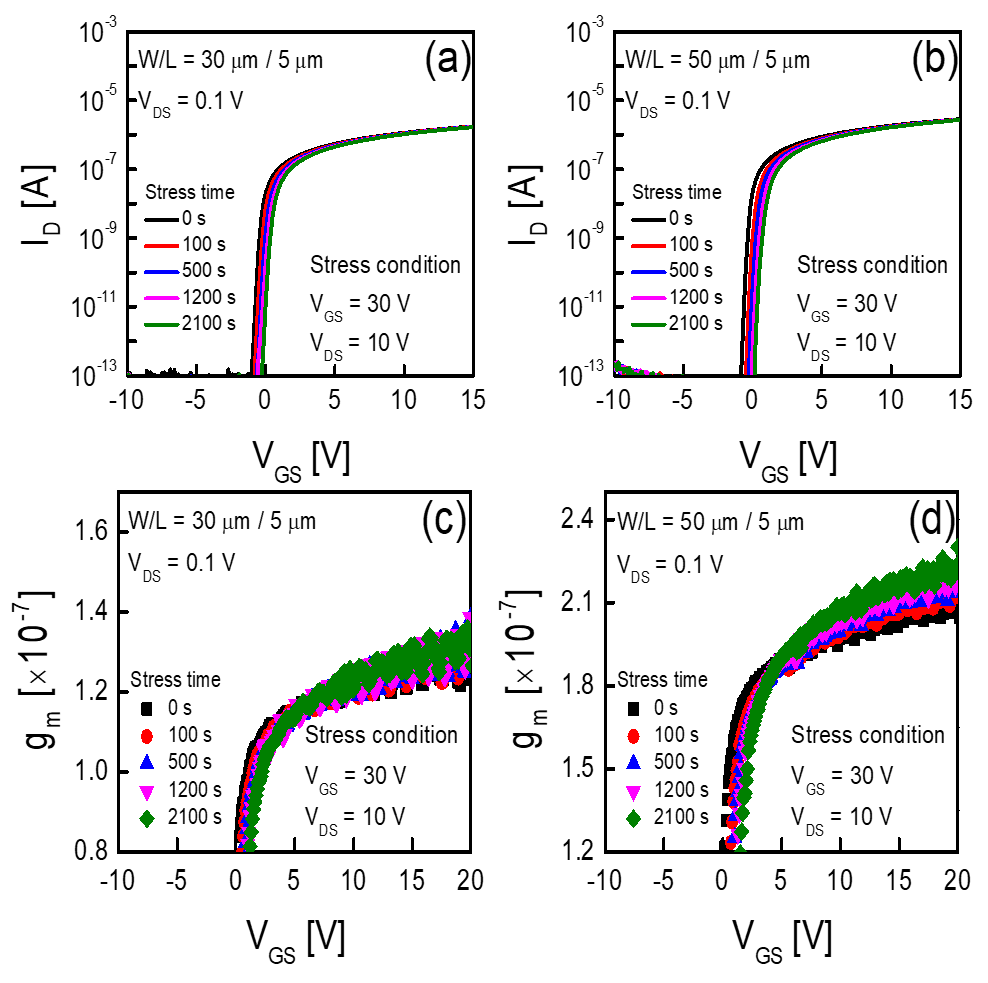##### Fig. 3. Time dependence of transfer curves in the saturation region (V$_{\mathrm{DS}}$ = 15 V) measured from the IGZO TFT with W/L = 30/5 ${\mu}$m in the (a) forward; (b) reverse modes under SHS. Time dependence of transfer curves in the saturation region (V$_{\mathrm{DS}}$ = 15 V) measured from the IGZO TFT with W/L = 50/5~${\mu}$m in the (c) forward; (d) reverse modes under SHS.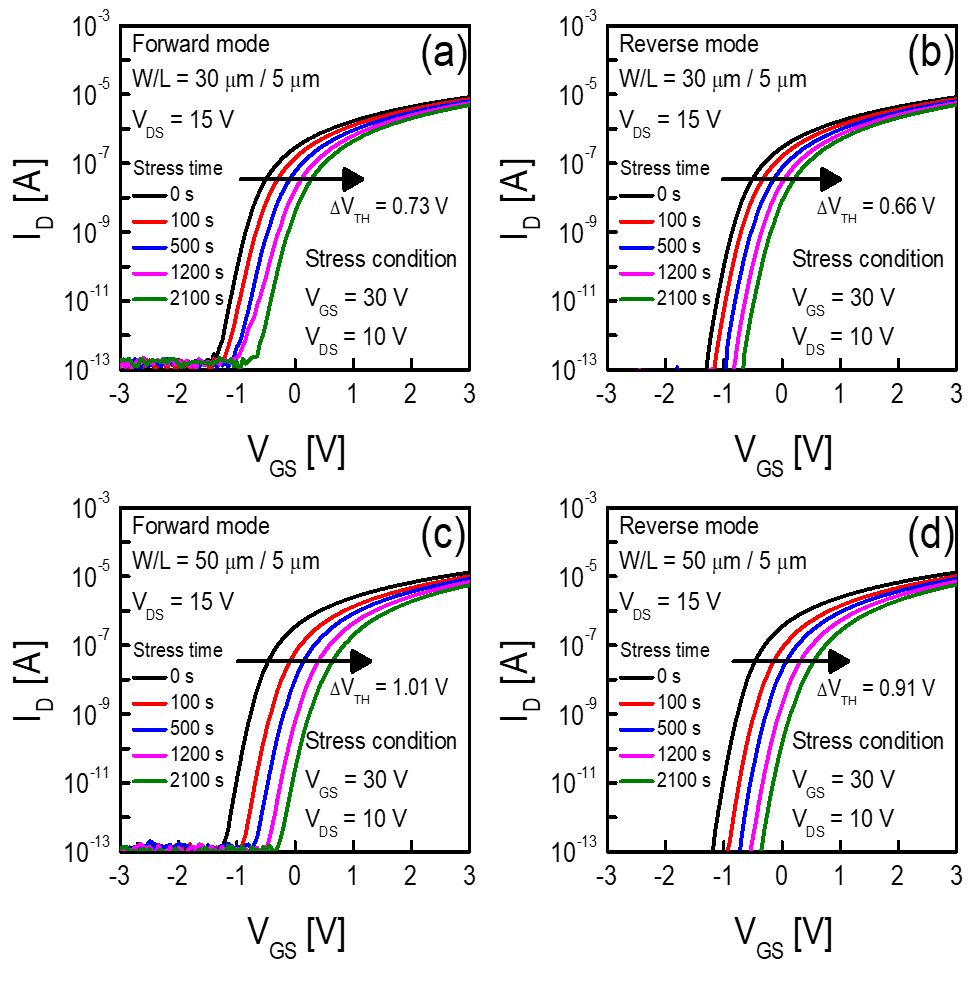##### Fig. 4. Small signal: (a) C$_{\mathrm{GS}}$-V$_{\mathrm{GS}}$; (b) C$_{\mathrm{GD}}$-V$_{\mathrm{GS}}$ curves measured at a frequency of 50 kHz from the IGZO TFT with W/L = 30/5 ${\mu}$m before and after application of SHS for 2100 s. Small signal; (c) C$_{\mathrm{GS}}$-V$_{\mathrm{GS}}$; (d) C$_{\mathrm{GD}}$-V$_{\mathrm{GS}}$ curves measured at a frequency of 50 kHz from the IGZO TFT with W/L = 50/5 ${\mu}$m before and after application of SHS for 2100 s.##### Fig. 5. Energy distribution of the subgap DOS extracted from IGZO TFTs with W/L = 30/5 ${\mu}$m and 50/5 ${\mu}$m near the (a) source; (b) drain sides of the IGZO TFT before and after application of SHS for 2100 s.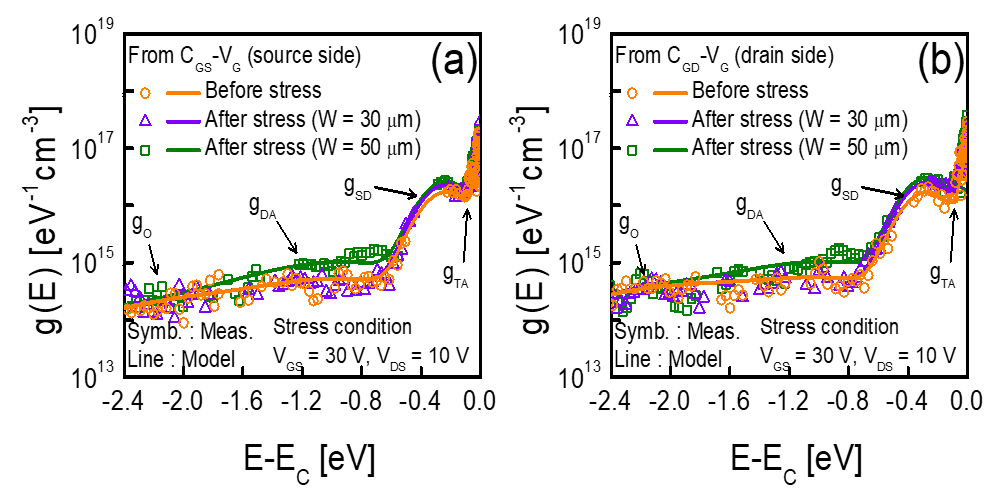##### Fig. 6. (a) Schematic illustration of the ${\Delta}$V$_{\mathrm{TH}}$ decomposition scheme based on the subgap DOS-based ${\Delta}$V$_{\mathrm{TH}}$ decomposition technique. The ${\Delta}$V$_{\mathrm{TH \_ SD}}$ and ${\Delta}$V$_{\mathrm{TH \_ DA}}$ values were extracted near the source and drain sides after applying SHS for 2100 s from IGZO TFTs with (b) W/L = 30/5 ${\mu}$m; (c) 50/5 ${\mu}$m.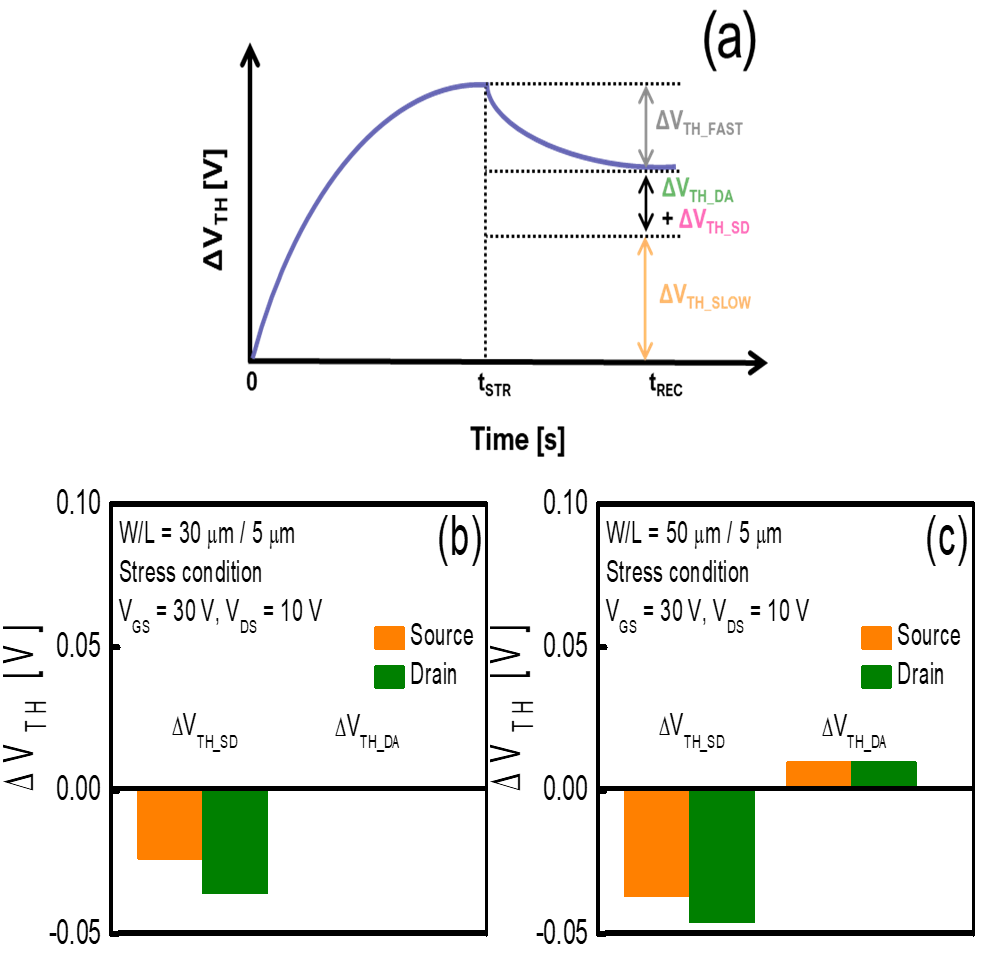##### Fig. 7. Time evolution of ${\Delta}$V$_{\mathrm{TH}}$ during SHS and recovery phases extracted during forward and reverse mode operation from IGZO TFTs with (a) W/L = 30/5 ${\mu}$m; (b) 50/5 ${\mu}$m. The ${\Delta}$V$_{\mathrm{TH}}$ values originated from each degradation mechanism after applying SHS for 2100 s extracted near the source and drain sides from IGZO TFTs with (a) W/L = 30/5 ${\mu}$m; (b) 50/5~${\mu}$m.## IV. CONCLUSIONS

In this study, we conducted a quantitative analysis of the effects of channel width on SHS-induced ${\Delta}$V$_{\mathrm{TH}}$ in IGZO TFTs. The analysis was conducted using TG-SA coplanar IGZO TFTs with different channel widths (W/L = 30/5 ${\mu}$m and 50/5 ${\mu}$m) and the SHS was applied under bias conditions of V$_{\mathrm{GS}}$ = 30 V and V$_{\mathrm{DS}}$ = 10 V. By applying the subgap DOS-based ${\Delta}$V$_{\mathrm{TH}}$ decomposition technique, we concluded that ${\Delta}$V$_{\mathrm{TH}}$ under SHS in the fabricated IGZO TFTs was caused by increases in g$_{\mathrm{SD}}$ and g$_{\mathrm{DA}}$ in the IGZO thin film and electron trapping in the fast and slow traps of the SiO$_{\mathrm{X}}$ gate dielectric. However, we also observed that every ${\Delta}$V$_{\mathrm{TH}}$ originating from each degradation mechanism increased with an increase in channel width. Moreover, we concluded that the increase in ${\Delta}$V$_{\mathrm{TH}}$_$_{\mathrm{SLOW}}$ due to the higher channel temperature was the dominant reason for more significant electrical performance degradation in the fabricated IGZO TFT with a wider channel width after SHS.

## ACKNOWLEDGMENTS

This research was supported by the Chung-Ang University Research Scholarship Grants in 2022, Samsung Display Co., Ltd. and the National Research Foundation of Korea (NRF) grant funded by the Korean government (MSIT) (2020R1A2B5B01001765).

## References

1
N. On et al., “Hot Carrier Effect in Self-Aligned In-Ga-Zn-O Thin-Film Transistors with Short Channel Length,” IEEE Transactions on Electronic Devices, Vol. 67, No. 12, pp. 5544-5551, Dec., 2020.2
H. J. Kim et al., “Channel defect analysis method of a-igzo tfts on polyimide for flexible displays,” Journal of Semiconductor Technology and Science, Vol. 20, No. 5, pp. 474-478, Oct., 2020.3
E. Fortunato, P. Barquinha, and R. Martins, “Oxide semiconductor thin-film transistors: A review of recent advances,” Advanced Materials, Vol. 24, No. 22. pp. 2945-2986, Jun., 2012.4
J. K. Jeong, “The status and perspectives of metal oxide thin-film transistors for active matrix flexible displays,” Semiconductor Science and Technology, Vol. 26, No. 3, Mar., 2011.5
J. Park et al., “Effect of Positive Bias Stress on the Back-Gate Voltage-Modulated Threshold Voltage in Double-Gate Amorphous InGaZnO Thin-Film Transistors,” IEEE Electron Device Letters, Vol. 43, No. 11, pp. 1878-1881, Nov., 2022.6
K. Abe, K. Ota, and T. Kuwagaki, “Simulation Study of Self-Heating and Edge Effects on Oxide-Semiconductor TFTs: Channel-Width Dependence,” in Proceedings of the International Display Workshops, Vol. 26, pp. 461-464. Nov., 2019.7
A. Perinot, M. Giorgio, and M. Caironi, “Flexible Carbon-based Electronics,” (Eds: P. Samorí, V. Palermo), Wiley-VCH, Weinheim, Germany, Vol. 71, Oct., 2018.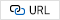8
M. Fujii et al., “Experimental and Theoretical Analysis of Degradation in Ga2O3 -In2O3 -ZnO Thin-Film Transistors,” Japanese Journal of Applied Physics, Vol. 48, No. 4, pp. 04C091, Apr., 2009.9
T.-C. Chen et al., “Self-heating enhanced charge trapping effect for InGaZnO thin film transistor,” Applied Physics Letters, Vol. 101, No. 4, pp. 042101, Jul., 2012.10
C.-Y. Jeong et al., “A study on the degradation mechanism of InGaZnO thin-film transistors under simultaneous gate and drain bias stresses based on the electronic trap characterization,” Semiconductor Science and Technology, Vol. 29, No. 4, pp. 045023, Apr., 2014.11
D. K. Seo et al., “Drastic improvement of oxide thermoelectric performance using thermal and plasma treatments of the InGaZnO thin films grown by sputtering,” Acta Materialia, Vol. 59, No. 17, pp. 6743-6750, Oct., 2011.12
J. Lee et al., “Modeling and Characterization of the Abnormal Hump in n-Channel Amorphous-InGaZnO Thin-Film Transistors After High Positive Bias Stress,” IEEE Electron Device Letters, Vol. 36, No. 10, pp. 1047-1049, Oct., 2015.13
D. Lee et al., “Asymmetrical degradation behaviors in amorphous InGaZnO thin-film transistors under various gate and drain bias stresses,” Journal of Vacuum Science & Technology B, Nanotechnology and Microelectronics: Materials, Processing, Measurement, and Phenomena, Vol. 33, No. 1, pp. 011202, Jan., 2015.14
K. Kise et al., “Self-heating induced instability of oxide thin film transistors under dynamic stress,” Applied Physics Letters, Vol. 108, No. 2, pp. 023501, Jan., 2016.15
H. Bae et al., “Extraction Technique for Intrinsic Subgap DOS in a-IGZO TFTs by De-Embedding the Parasitic Capacitance Through the Photonic C-V Measurement,” IEEE Electron Device Letters, Vol. 34, No. 1, pp. 57-59, Jan., 2013.16
H. Bae et al., “Single-Scan Monochromatic Photonic Capacitance-Voltage Technique for Extraction of Subgap DOS Over the Bandgap in Amorphous Semiconductor TFTs,” IEEE Electron Device Letters, Vol. 34, No. 12, pp. 1524-1526, Dec., 2013.17
S. Lee et al., “Extraction of Subgap Density of States in Amorphous InGaZnO Thin-Film Transistors by Using Multifrequency Capacitance-Voltage Characteristics,” IEEE Electron Device Letters, Vol. 31, No. 3, pp. 231-233, Mar., 2010.18
B.-S. Yeh, “Modeling and characterization of amorphous oxide semiconductor thin-film transistors,” Ph.D. dissertation, Dept. Electr. Eng. Comput. Sci., Oregon State Univ., Corvallis, OR, USA, 2015.19
M. Mativenga et al., “Origin of light instability in amorphous IGZO thin-film transistors and its suppression,” Scientific Reports, Vol. 11, No. 1, pp. 14618, Dec., 2021.20
J. Park et al., “Numerical Analysis on Effective Mass and Traps Density Dependence of Electrical Characteristics of a-IGZO Thin-Film Transistors,” Electronics, Vol. 9, No. 1, pp. 119, Jan., 2020.21
S. Choi et al., “Effect of Oxygen Content on Current Stress-Induced Instability in Bottom-Gate Amorphous InGaZnO Thin-Film Transistors,” Materials, Vol. 12, No. 19, pp. 3149, Sep., 2019.22
S. Choi et al., “Influence of the Gate/Drain Voltage Configuration on the Current Stress Instability in Amorphous Indium-Zinc-Oxide Thin-Film Transistors With Self-Aligned Top-Gate Structure,” IEEE Electron Device Letters, Vol. 40, No. 9, pp. 1431-1434, Jul., 2019.23
S.-I. Oh, J.-M. Woo, and J.-H. Jang, “Comparative Studies of Long-Term Ambiance and Electrical Stress Stability of IGZO Thin-Film Transistors Annealed Under Hydrogen and Nitrogen Ambiance,” IEEE Transactions on Electron Devices, Vol. 63, No. 5, pp. 1910-1915, May, 2016.24
X. Zhang et al., “P-1.6: Effect of Deposition Condition of Passivation Layer on the Performance of Self-Aligned Top-Gate a-IGZO TFTs,” SID Symposium Digest of Technical Papers, Vol. 49, pp. 535-537, Apr., 2018.25
X. D. Huang et al., “Effects of Metal-Hydroxyl and InOx Defects on Performance of InGaZnO Thin-Film Transistor,” IEEE Transactions on Electron Devices, Vol. 65, No. 3, pp. 1009-1013, Mar., 2018.26
H. Noh et al., “Role of Hydrogen in Active Layer of Oxide-Semiconductor-Based Thin Film Transistors,” Crystals, Vol. 9, No. 2, pp. 75, Jan., 2019.27
M. M. Sabri et al., “Hydroxyl radical-assisted decomposition and oxidation in solution-processed indium oxide thin-film transistors,” Journal of Materials Chemistry C, Vol. 3, No. 28, pp. 7499-7505, Jul., 2015.28
D. H. Kim et al., “Experimental decomposition of the positive bias temperature stress-induced instability in self-aligned coplanar InGaZnO thin-film transistors and its modeling based on the multiple stretched-exponential functions,” Journal of the Society for Information Display, Vol. 25, No. 2, pp. 98-107, Feb., 2017.29
S. Choi et al., “Systematic Decomposition of the Positive Bias Stress Instability in Self-Aligned Coplanar InGaZnO Thin-Film Transistors,” IEEE Electron Device Letters, Vol. 38, No. 5, pp. 580-583, May, 2017.30
G. Li et al., “Understanding hydrogen and nitrogen doping on active defects in amorphous In-Ga-Zn-O thin film transistors,” Applied Physics Letters, Vol. 112, No. 25, pp. 253504, Jun., 2018.31
J. Rhee et al., “The electron trap parameter extraction-based investigation of the relationship between charge trapping and activation energy in IGZO TFTs under positive bias temperature stress,” Solid-State Electronics, Vol. 140, pp. 90-95, Feb., 2018.32
D.-H. Kim et al., “Quantitative Analysis of Positive-Bias-Stress-Induced Electron Trapping in the Gate Insulator in the Self-Aligned Top Gate Coplanar Indium-Gallium-Zinc Oxide Thin-Film Transistors,” Coatings, Vol. 11, No. 10, pp. 1192, Sep., 2021.Dong-Ho Lee received the B.S degree in electronic engineering from Gachon University, Gyeonggi-Do, South Korea, in 2020. Since 2020, He is currently pursuing the integrated M.S., Ph.D. degrees in electrical and electronics engineering from Chung-Ang University. His current research interest includes the reliability study of oxide thin-film transistors.

Hwan-Seok Jeong received the B.S. degree in chemistry from Dae-Jin University, Pochen, South Korea, in 2015. He is currently pursuing the Ph.D. degree in electrical and electronics engineering from Chung-Ang University. His current research interest includes the fabrication and reliability study of oxide thin-film transistors.

Yeong-Gil Kim received the B.S. degree in electronic engineering from Seoul National University of Science and Technology, Seoul, South Korea, in 2022. He is currently pursuing the M.S. degree in electrical and electronics engineering from Chung-Ang University. His current research interest includes the fabrication and reliability study of oxide thin-film transistors.

##### Myeong-Ho Kim

Myeong-Ho Kim is a research engineer with the Research and Development Center, Samsung Display, Yongin, South Korea.

##### Kyoung Seok Son

Kyoung Seok Son is a research engineer with the Research and Development Center, Samsung Display, Yongin, South Korea.

##### Jun Hyung Lim

Jun Hyung Lim received the Ph.D. degree from the Department of Materials Science and Engineering, Sungkyunkwan University, Suwon, South Korea, in 2006. He is in charge of the oxide backplane with the Research and Development Center, Samsung Display, Yongin, South Korea.

Sang-Hun Song received his BS degree in Electronics Engineering from Seoul National University in 1886 and his MA and Ph.D. degrees from Princeton University in 1988 and 1997, respectively. His doctoral research studies on magneto-optical and magneto-transport properties of the 2- dimensional carriers in strained semiconductor layers. In 1997, he joined LG Semicon Co. Ltd. As a DRAM circuit designer. In 2001, he joined the School of Electrical and Electronics Engineering at Chung-Ang University in Seoul, where his now a professor. His research interests include semiconductor materials and devices, and their applications to real world electronic systems.

Hyuck-In Kwon received the B.S., M.S., and Ph.D. degrees in electrical engineering from Seoul National University, Seoul, South Korea, in 1999, 2001, and 2005, respectively. From August 2004 to March 2006, he was a Research Associate with the University of Illinois at Urbana-Champaign. In 2006, he joined the System LSI Division, Samsung Electronics Company, South Korea, where he was a Senior Engineer with the Image Development Team. From September 2007 to February 2010, he was with the School of Electronic Engineering, Daegu University, as a full-time Lecturer and an Assistant Professor. Since 2010, he has been with Chung-Ang University, Seoul, where he is currently a Professor with the School of Electrical and Electronics Engineering. His research interests include CMOS active pixel image sensors, oxide thin-film transistors, and silicon nanotechnologies.